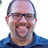Foundation

## Foundation Forum

##### multiple equalizer in one container
• 1

Hi,
can I insert multiple equalizer in one container?

Something like this:
(I had tried with data-equalizer="ONE" data-equalizer="TWO" and data-equalizer="ONE,TWO" but it doesn't work)

I'd like to get as final result his kind of scenario: all bottom elements with the same height and all top elements with another same height.

``````<div class="row" data-equalizer="ONE" data-equalizer="TWO">
<div class="medium-4">
<div class="top" data-equalizer-watch="ONE"></div>
<div class="bottom" data-equalizer-watch="TWO"></div>
</div>
<div class="medium-4">
<div class="top" data-equalizer-watch="ONE"></div>
<div class="bottom" data-equalizer-watch="TWO"></div>
</div>
<div class="medium-4">
<div class="top" data-equalizer-watch="ONE"></div>
<div class="bottom" data-equalizer-watch="TWO"></div>
</div>
</div>``````

Thanks

• 1

Hi,
can I insert multiple equalizer in one container?

Something like this:
(I had tried with data-equalizer="ONE" data-equalizer="TWO" and data-equalizer="ONE,TWO" but it doesn't work)

I'd like to get as final result his kind of scenario: all bottom elements with the same height and all top elements with another same height.

``````<div class="row" data-equalizer="ONE" data-equalizer="TWO">
<div class="medium-4">
<div class="top" data-equalizer-watch="ONE"></div>
<div class="bottom" data-equalizer-watch="TWO"></div>
</div>
<div class="medium-4">
<div class="top" data-equalizer-watch="ONE"></div>
<div class="bottom" data-equalizer-watch="TWO"></div>
</div>
<div class="medium-4">
<div class="top" data-equalizer-watch="ONE"></div>
<div class="bottom" data-equalizer-watch="TWO"></div>
</div>
</div>``````

Thanks

This post has been closed. No new replies can be added.

#####Corey Schaafover 3 years ago

Andrea,

It's not possible to put the data-equalizer on the same element. I would recommend changing your markup as it's just a nesting issue.

You could try something along these lines:

``````<div class="row" data-equalizer="ONE">
<div class="medium-12">
<ul class="row" data-equalizer="TWO">
<li class="medium-4">
<div class="top" data-equalizer-watch="ONE"></div>
<div class="bottom" data-equalizer-watch="TWO"></div>
</li>
<li class="medium-4">
<div class="top" data-equalizer-watch="ONE"></div>
<div class="bottom" data-equalizer-watch="TWO"></div>
</li>
<li class="medium-4">
<div class="top" data-equalizer-watch="ONE"></div>
<div class="bottom" data-equalizer-watch="TWO"></div>
</li>
</ul>
</div>
</div>``````